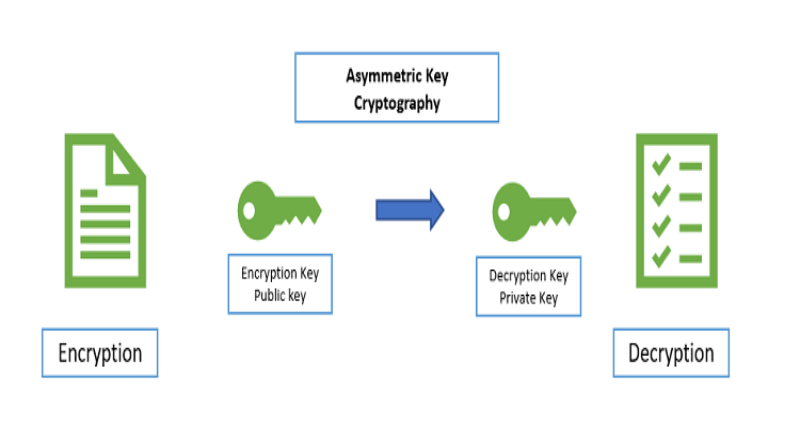Asymmetric cryptography is one of the most potent computer encryption methods, based on a relatively sophisticated mathematical formula to generate a pair of keys: the private and public keys. Both the sender and the receiver must employ asymmetric cryptography with the same technique to develop a unique and unrepeatable set of keys for each other

The sender and receiver provide their public keys throughout this communication process, which will then use to encrypt the communications they transmit and receive. And the private keys will decode the contacts to see what they contain. This technique prevents a third party from interfering with the transmission or knowing the letter range.

This approach, which appears simple but conceals years of study and sophisticated algorithms, is widely utilized. May found asymmetric encryption in our WhatsApp or Telegram chats, as well as in our email access.

## Beginnings Of Asymmetric Cryptography

Asymmetric cryptography saw its birth in 1976, when Ralph Merkel, Whitfield Diffie, and Martin Hellman created the first public asymmetric cryptography system. Although not precisely asymmetric, the Diffie-Hellman algorithm was a crucial first step in developing this technique, which soon saw more contributions from other researchers.

It would be in 1977 when Ron Rivest, Adi Shamir, and Leonard Adleman created the RSA algorithm. This first public asymmetric cryptography algorithm would become an industry standard to this day, where the evolution of technology has allowed the further development of this encryption technique.

## How Does Asymmetric Cryptography Work?

The operation of asymmetric encryption follows a series of steps that guarantee that the system is entirely secure and maintains the secrecy of the communications exchanged through it.

## Select The Encryption Algorithm And Curve

The first step for asymmetric encryption to work is to choose the algorithm to use because each has unique properties. There are currently dozens of algorithms, some more secure than others depending on their complexity, but the most common are RSA, ECDSA, EdDSA, and ElGamal; each of these algorithms uses its mathematical system.

## Asymmetric Cryptography – Key GenerationOnce the algorithm has been decided, the next step is to generate the public and private keys.

The first key to being generated using this cryptography technique is the private key. We only have this key, and it arises as to the result of taking random data and transferring it to a mathematical problem. A considerable number is obtained after going through a conversion process

We can generate the public key through a complex mathematical process that relates the private key to a mathematical formulation with the private key. Again, it will transform a considerable number into a long string of numbers and letters as the public key.

Thus, the private key is an encryption seed, from which we can create as many public keys as we need. The private key also decrypts the messages we receive from the people we give our public keys. In addition, the private key allows us to digitally sign the letters. Thus confirming the authenticity of the communication since no one can duplicate the private key.

For its part, the purpose of the public key is that the people to whom we give it can encrypt the messages and send them to us. It is essential to point out that the public key allows messages to be encrypted. But the opposite process, decrypting them, is practically impossible. It is so because the private and public keys will relate to the algorithm used to create them. The public key also verifies the authenticity of the digital signatures that our messages carry.

• It has a high-security rate since the encryption scheme is very complex; this makes cryptanalysis difficult and brute force attacks to break it useless.
• Thanks to public and private vital sets, it ensures open and public communication channels.
• It allows authenticating the information thanks to a digital signature system. For example, in the FNMT certificates, a system without encrypted critical repudiation will use
• They have a high level of confidentiality and integrity.